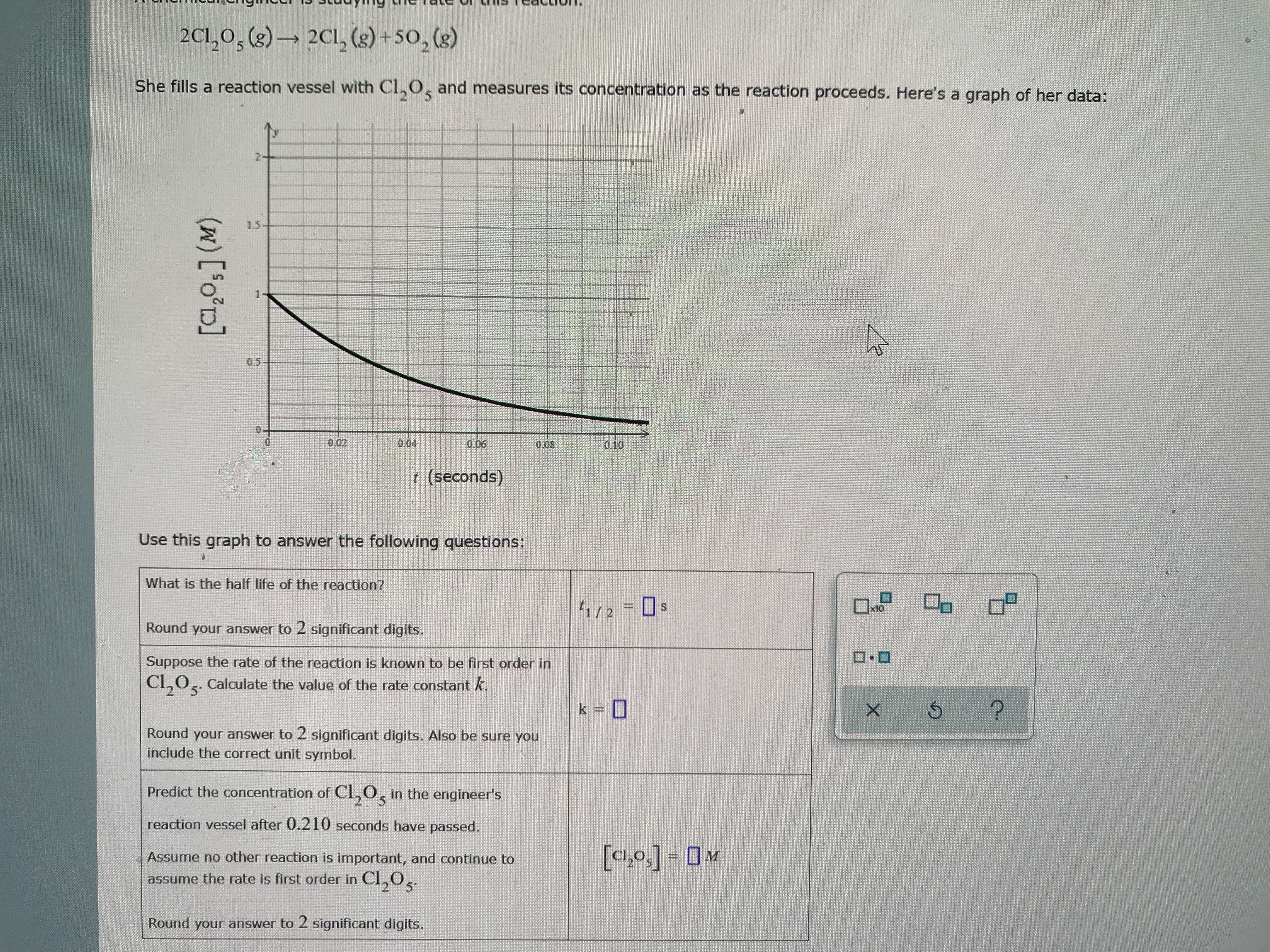# 2C1,0s (8)2Cl, (s)+50, (s)She fills a reaction vessel with Cl,O, and measures its concentration as the reaction proceeds. Here's a graph of her data:2-151-050.020.040.0S010r (seconds)Use this graph to answer the following questions:What is the half life of the reaction?f1/2Round your answer to 2 significant digits.Suppose the rate of the reaction is known to be first order in|C20. Calculate the value of the rate constant k.k?Round your answer to 2 significant digits. Also be sure youinclude the correct unit symbol.Predict the concentration of CL,0, in the engineer's5reaction vessel after 0.210 seconds have passed.[a,o]Assume no other reaction is important, and continue toMassume the rate is first order in Cl0Round your answer to 2 significant digits.(w) ['o'D]

Question
12 views

What is the half-life of this reaction. calculate the value of the rate constant K. predict the concentration of Cl2O5 in the engineers reaction vessel after .210 seconds have passed. Please round for answers only. 2 sig figs. Please check work. Thank youhelp_outlineImage Transcriptionclose2C1,0s (8) 2Cl, (s)+50, (s) She fills a reaction vessel with Cl,O, and measures its concentration as the reaction proceeds. Here's a graph of her data: 2- 15 1- 05 0.02 0.04 0.0S 010 r (seconds) Use this graph to answer the following questions: What is the half life of the reaction? f1/2 Round your answer to 2 significant digits. Suppose the rate of the reaction is known to be first order in |C20. Calculate the value of the rate constant k. k ? Round your answer to 2 significant digits. Also be sure you include the correct unit symbol. Predict the concentration of CL,0, in the engineer's 5 reaction vessel after 0.210 seconds have passed. [a,o] Assume no other reaction is important, and continue to M assume the rate is first order in Cl0 Round your answer to 2 significant digits. (w) ['o'D] fullscreen
check_circle

Step 1

Part 1:

According to the definition, half life is the time needed to reduce the concentration of the reactant to half of its initial value.

Therefore from the graph, the half life of the reaction...

### Want to see the full answer?

See Solution

#### Want to see this answer and more?

Solutions are written by subject experts who are available 24/7. Questions are typically answered within 1 hour.*

See Solution
*Response times may vary by subject and question.
Tagged in

### Chemical Kinetics